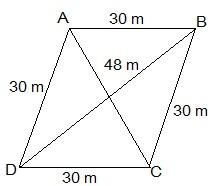# A rhombus shaped field has green grass for 18 cows to graze. If each side of the rhombus is 30 m and its longer diagonal is 48 m, how much area of grass field will each cow be getting?Given

ABCD is a rhombus having diagonal AC = 48 cm

The diagonal of the Rhombus divides the area into two equal parts.

Find out

We have to determine how much area of grass field will each cow be getting

Solution

Using Heron’s formula, area of the triangle =$$\sqrt{s(s-a)(s-b)(s-c)}$$

Now, ar(△ABC) can be calculated by Heron’s Formula, where

AB = a = 30 m

BC = b = 30 m

AC = c = 48 m

Semi Perimeter (s) = a+b+c/2

s = 30+38+48/2

s = 54 m

ar(△ABC) = √s(s-a)(s-b)(s-c)

= √54(54-30)(54-30)(54-48)

= √54×(24)×(24)×(6)

= 432 m2

Hence, Area of rhombus = 2 × (ar(△))

= 2 × 432 = 864 m2

Area for 18 cows = 864 m2

Area for each cow = 864 / 18 = 48 m2